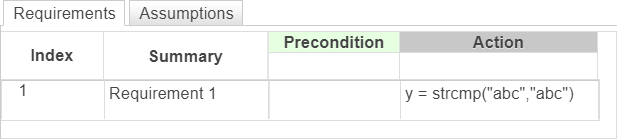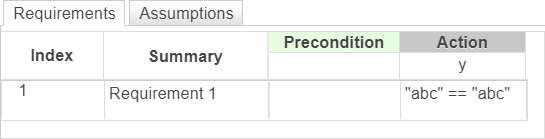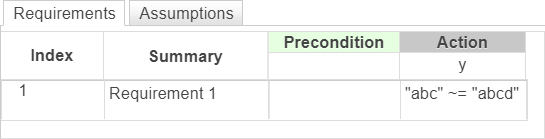# strcmp

Compare strings (case sensitive)

Since R2022b

## Syntax

``tf = strcmp(str1,str2)``

## Description

example

````tf = strcmp(str1,str2)` compares the strings `str1` and `str2`. The operator returns `1` (`true`) if the strings are identical, and returns `0` (`false`) otherwise. `strcmp` is case sensitive. Use this operator in the Requirements Table block.```

## Examples

expand all

In a Requirements Table block, create a requirement that checks if the strings `"abc"` and `"abc"` are equal.

`y = strcmp("abc","abc")`You can also compare and sort string with relational operators. Use `==` to determine two strings are equal.

`"abc" == "abc"`Use `~=` to determine if two strings are not equal.

`"abc" ~= "abcd"`## Version History

Introduced in R2022b